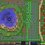# IIT JEE 1982 - 'Adapted' - Mathematics Question Paper

IIT JEE 1982 Mathematics 'Adapted' Question Paper

If you are attempting this as a test:

Time: 2 hours

Maximum Marks: 154

Go the IIT way - get it right in first attempt, or attempt afterwards during test analysis, or see solution if available. You may even note your answer on a piece of paper. And then enter and check all of them after the time ends.

Paper Pattern

1. There are 14 single correct type questions, with +3 marks for each correct answer and -1 mark for each wrong answer.

2. There are 8 single-digit integer type questions, with +3 marks for each correct answer and -1 mark for each wrong answer. The answer to each is a single digit integer from 0 to 9 (both inclusive).

3. There are 22 multiple correct answers type questions, with +4 marks for each correct answer and -1 for each wrong answer.

Marking Scheme

1. (+3, -1) each for Single Correct and Single-Digit Integer. [The answer to Single-Digit Integer is an integer from 0 to 9 (both inclusive).]

2. (+4, -1) each for Multi-Correct

:provided you are attempting only once.

Actual Subjective Questions appear at the bottom of this Set in the form of a Note. You are left to your own devices with regards to honesty, wherever 'Show-that' questions appear.Note by Shubhamkar Ayare
4 years, 3 months ago

This discussion board is a place to discuss our Daily Challenges and the math and science related to those challenges. Explanations are more than just a solution — they should explain the steps and thinking strategies that you used to obtain the solution. Comments should further the discussion of math and science.

When posting on Brilliant:

• Use the emojis to react to an explanation, whether you're congratulating a job well done , or just really confused .
• Ask specific questions about the challenge or the steps in somebody's explanation. Well-posed questions can add a lot to the discussion, but posting "I don't understand!" doesn't help anyone.
• Try to contribute something new to the discussion, whether it is an extension, generalization or other idea related to the challenge.

MarkdownAppears as
*italics* or _italics_ italics
**bold** or __bold__ bold
- bulleted- list
• bulleted
• list
1. numbered2. list
1. numbered
2. list
Note: you must add a full line of space before and after lists for them to show up correctly
paragraph 1paragraph 2

paragraph 1

paragraph 2

[example link](https://brilliant.org)example link
> This is a quote
This is a quote
    # I indented these lines
# 4 spaces, and now they show
# up as a code block.

print "hello world"
# I indented these lines
# 4 spaces, and now they show
# up as a code block.

print "hello world"
MathAppears as
Remember to wrap math in $$ ... $$ or $ ... $ to ensure proper formatting.
2 \times 3 $2 \times 3$
2^{34} $2^{34}$
a_{i-1} $a_{i-1}$
\frac{2}{3} $\frac{2}{3}$
\sqrt{2} $\sqrt{2}$
\sum_{i=1}^3 $\sum_{i=1}^3$
\sin \theta $\sin \theta$
\boxed{123} $\boxed{123}$

Sort by:

Thanks a lot!

- 2 years, 4 months ago